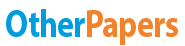# Economics Expenditure Multiplier

Essay by   •  June 22, 2019  •  Course Note  •  342 Words (2 Pages)  •  258 Views

## Essay Preview: Economics Expenditure Multiplier

Report this essay
Page 1 of 2

Chapter (4) –

Exercise: Consider the following table

 National income (Y) Consumption (C) Investment (I) Government spending (G) Aggregate expenditure (AE) 0 120 15 25 100 180 200 240 300 300 400 360 500 420 600 480 700 540 800 600
 1 The value of autonomous consumption is 2 The value of autonomous investment is 3 The value of autonomous government spending is 4 The value of autonomous expenditure is 5 The value of MPC 6 The value of MPS 7 Consumption function is 8 Saving function is 9 The value of equilibrium consumption is 10 The value of equilibrium expenditure is 11 The size of multiplier is 12 What is the value of unplanned inventory when national income (y) = 700 13 Find new equilibrium expenditure when autonomous expenditure increase by \$20 14 Find new equilibrium expenditure when autonomous expenditure decrease by \$20 15 Draw AE curve, label your graph, show the equilibrium expenditure

Chapter (4) –

 National income (Y) Consumption (C) Investment (I) Government spending (G) Aggregate expenditure (AE) Unplanned inventory UI The country should 0 120 15 25 160 -160 Increase GDP 100 180 15 25 220 -120 Increase GDP 200 240 15 25 280 -80 Increase GDP 300 300 15 25 340 -40 Increase GDP 400 360 15 25 400 0 No change 500 420 15 25 460 40 Decrease GDP 600 480 15 25 520 80 Decrease GDP 700 540 15 25 580 120 Decrease GDP 800 600 15 25 640 160 Decrease GDP

...

...